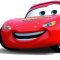## Number of arrays of size N whose elements are positive integers and sum is K in C++Hafeezul Kareem

Updated on 26-Oct-2021 06:33:42

We are given two numbers n and k. We need to find the count of arrays that can be formed using the n numbers whose sum is k.The number of arrays of size N with sum K is \$\dbinom{k - 1}{n - 1}\$.This a straightforward formula to find the number ... Read More

## Number of anomalies in an array in C++Hafeezul Kareem

Updated on 26-Oct-2021 06:03:10

In this tutorial, we are going to write a program that finds the number of anomalies in the given array.A number is an anomaly in the given array if the absolute difference between the number and all other numbers is greater than the given number k. Let's see an example.Inputarr ... Read More

## Number is divisible by 29 or not in C++Hafeezul Kareem

Updated on 26-Oct-2021 06:00:45

It's a straightforward problem. We can use the modulo (%) operator to check whether the given number is divisible by 29 or not. Let's see some examples.Input29 254Output1 0AlgorithmImplementationFollowing is the implementation of the above algorithm in C++#include <bits/stdc++.h> using namespace std; bool isDivisibleBy29(long long n) {    return n ... Read More

## Multiply two polynomials in C++Hafeezul Kareem

Updated on 26-Oct-2021 05:56:45

The coefficients of each term of the polynomial are given in an array. We need to multiply the two polynomials. Let's see an example.InputA = [1, 2, 3, 4] B = [4, 3, 2, 1]Output4x6 + 11x5 + 20x4 + 30x3 + 20x2 + 11x1 + 4AlgorithmInitialise two polynomials.Create a ... Read More

## Multiply two numbers represented as linked lists into a third list in C++Hafeezul Kareem

Updated on 25-Oct-2021 17:04:14

Given two linked lists with digits in it. We need to multiply two numbers formed by the linked list. It can be done easily by forming the numbers from the two linked lists. Let's see an example.Input1 -> 2 -> NULL 2 -> 3 -> NULLOutput2 -> 7 -> 6 ... Read More

## Multiply two numbers represented by Linked Lists in C++Hafeezul Kareem

Updated on 25-Oct-2021 16:28:34

Given two linked lists with digits in it. We need to multiply two numbers formed by the linked list. It can be done easily by forming the numbers from the two linked lists. Let's see an example.Input1 -> 2 -> NULL 2 -> 3 -> NULLOutput2 -> 7 -> 6 ... Read More

## Multiply Large Numbers represented as Strings in C++Hafeezul Kareem

Updated on 25-Oct-2021 07:10:44

Given two numbers in the string formats. We need to multiply them. The idea to solve the problem is to maintain a previous digit multiplication answer and carry. We can use the previous digits multiplication answer and carry to get the next set digits multiplication.Let's see an example.Input15 2Output30AlgorithmInitialise the ... Read More

## Multiply any Number with using Bitwise Operator in C++Hafeezul Kareem

Updated on 25-Oct-2021 06:31:30

In this tutorial, we are going write a program that multiplies the given two numbers using bitwise operators.The left shift (<<) operator is used for the multiplication whereas the right shift (>>) is used for the division.The multiplication of two numbers x, y can be written as x * y ... Read More

## Multiply a number by 15 without using * and / operators in C++Hafeezul Kareem

Updated on 25-Oct-2021 06:23:26

We can using left shift (<<) operator to multiply with 15. If we left shift 1, then we are multiplying it with 2.If we left shift the given number with 4, then we will get the 16 * n. Subtracting the given number from 16 * n will result in ... Read More

## Multiply a given Integer with 3.5 in C++Hafeezul Kareem

Updated on 25-Oct-2021 05:48:06

To get the result of n * 3.5 we need to calculate (n * 2) + n + (n / 2). Moving the bits to left by 1 will give you n * 2 and moving the bits to right by will you n / 2. Add those to get ... Read More